GMAT  >  Test: Percentages- 1

# Test: Percentages- 1

Test Description

## 15 Questions MCQ Test Quantitative for GMAT | Test: Percentages- 1

Test: Percentages- 1 for GMAT 2023 is part of Quantitative for GMAT preparation. The Test: Percentages- 1 questions and answers have been prepared according to the GMAT exam syllabus.The Test: Percentages- 1 MCQs are made for GMAT 2023 Exam. Find important definitions, questions, notes, meanings, examples, exercises, MCQs and online tests for Test: Percentages- 1 below.
Solutions of Test: Percentages- 1 questions in English are available as part of our Quantitative for GMAT for GMAT & Test: Percentages- 1 solutions in Hindi for Quantitative for GMAT course. Download more important topics, notes, lectures and mock test series for GMAT Exam by signing up for free. Attempt Test: Percentages- 1 | 15 questions in 30 minutes | Mock test for GMAT preparation | Free important questions MCQ to study Quantitative for GMAT for GMAT Exam | Download free PDF with solutions
 1 Crore+ students have signed up on EduRev. Have you?
Test: Percentages- 1 - Question 1

### What is 1/10 % of 50?

Detailed Solution for Test: Percentages- 1 - Question 1

⇒ 1/10 % of 50

= 1/10 x 1/100 x 50

= 0.05

Test: Percentages- 1 - Question 2

### Find the value of y % of x? x = 5 x % of y = 2

Test: Percentages- 1 - Question 3

### Which of the following statements are true? I. If a positive number X is increased by 20% the resulting number would be 1.2X II.If a positive number X is decreased by 20 % the resulting number would be 0.8X III.32% of 24 is less than 24% of 32.

Detailed Solution for Test: Percentages- 1 - Question 3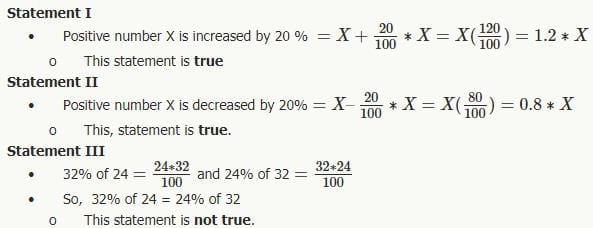Test: Percentages- 1 - Question 4

A positive number Y is first increased by 10% then decreased by 50% and then increased by 100%. The series of successive changes to Y is equivalent to a single change of

Detailed Solution for Test: Percentages- 1 - Question 4

Let the number be 100
10% of 100 is 10 = 110 (new Number)
50% of 110 is 55 = 110-55 = 55 (New Number)
100% of 55 is 55= 55+55= 110 (Final Number)
Final Number- Initial Number =110-100=10
And 10 is 10% of 100

Test: Percentages- 1 - Question 5

Restaurant A and Restaurant B decides to roll out a new plan which they believe will get them new customers. Restaurant A decides to give a 10% discount on the original bill amount, and then applies 15% tax on the discounted bill. Restaurant B, on the other hand applies a 15% tax on the original bill amount and then gives a 10% discount on the taxed bill.

If the original bill amount is same for both restaurants, which of the following must be true?

Detailed Solution for Test: Percentages- 1 - Question 5

Let the billing amount is 100

Case 1

10% discount on 100 = 10,
So 90 is the taxable amount
15% tax on 90= 13.5.
So total Bill = 90+13.5= 103.5 for Restaurant A

Case 2

15% tax on 100= 115
10 % discount on this is 11.5
Total Bill= 115-11.5= 103.5 for Restaurant B
Hence same in both case

Test: Percentages- 1 - Question 6

If 10 is 5 percent of 10 percent of a number, what is 25% of this number?

Detailed Solution for Test: Percentages- 1 - Question 6

Step 1: Let the number be x.
Therefore 10% of x = (10/100)*x = 0.1*x
5% of (10% of x) = (5/100)*0.1*x = 0.05*0.1*x = 0.005*x
Given that this value is equal to 10.

Step 2: Finding required values
Therefore,
0.005*x = 10

⇒ x = (10/0.005) = (10000/5)
⇒ x = 2000

Therefore, 25% of x = (1/4)*2000 = 500.

Test: Percentages- 1 - Question 7

Karen bought items A, B and C from a supermarket for a total price of \$1500. What was the price of item C?

(1) The money she spent to buy item A was 140% of the money she spent to buy the other two items

(2) Item B was 75% less expensive than item C

Detailed Solution for Test: Percentages- 1 - Question 7

Steps 1 & 2: Understand Question and Draw Inferences

Let the prices of the items A, B and C be a, b and c dollars respectively.

Given: a + b + c = 1500  . . . Equation 1

We need to find the value of c.

Step 3: Analyze statement 1

As per Statement 1:

The money she spent to buy item A was 140% of the money she spent to buy the other two items

a=140%of(b+c)a=140/100(b+c)a=7/5(b+c)...Equation2

By substituting Equation 2 in Equation 1, we will be able to determine the value of (b + c).

But we will not be able to find the value of c alone.

Therefore, Statement 1 is not sufficient.

Step 4: Analyze statement 2

As per Statement 2:

Item B was 75% less expensive than item C

b = c–75 % of c

b = c−75/100c

b=c/4.........Equ3

By substituting Equation 3 in Equation 1, we will get an expression in terms of a and c. We will not be determine a unique value for c.

Therefore, Statement 2 is not sufficient.

Step 5: Analyze Both Statements Together (if needed)

From Statement 1:

a = 7(b + c)/5     . . . Equation 2

From Statement 2:

b = c/4              . . . Equation 3

Substituting Equation 2 in Equation 1:

7(b + c)/5 + b + c = 1500

12(b + c)/5 =1500

Solving this equation, we get:
b + c = 625         . . . Equation 4

Substituting Equation 3 in Equation 4:

(c/4 + c) = 625

5c = 625 x 4

c = 500

Thus, we are able to get a unique value of c by using both the statements together.

Therefore, the correct answer is Option C.

Test: Percentages- 1 - Question 8

Tom’s salary has increased by 10% to \$11,000. Mike’s salary has reduced by 5%. What is Mike’s current salary if his old salary was two times Tom’s old salary?

Detailed Solution for Test: Percentages- 1 - Question 8

Step 1: Question statement and Inferences

We are given that the salary of Tom has increased by 10%, and now it has become \$11,000, whereas the salary of Mike has decreased by 5%.

It is also given that the old salary of Mike was twice that of Tom's old salary.

Let Tom’s old salary = TO

Let Mike’s old salary = MO and Mike’s new salary = MN

We need to find the value of MN

Step 2: Finding required values

TO + 10% of TO = 11000

⇒ 1.1* TO = 11000

⇒ TO = 10000

Therefore Tom’s old salary = \$10,000

It is also given that Mike’s old salary is twice Tom’s old salary.

⇒ MO = 2 * TO = 20000

Mike’s current salary is 5% less than his old salary.

MN = MO – 5% of MO

⇒ MN = 0.95*MO

⇒ MN = 0.95 * 20000 = 19000

Therefore Mike’s current salary = \$19,000

Test: Percentages- 1 - Question 9

During a housing slump, the number of houses for sale increases by 50% every month. What is the percentage increase in the number of houses on sale after 3 months?

Detailed Solution for Test: Percentages- 1 - Question 9

Step 1: Question statement and Inferences

We are given that during a housing slump, the number of houses for sale increases by 50% every month.

Let the initial number of houses for sale = x

We need to find the number of houses for sale at the end of 3 months to be able to calculate the required percentage increase.

Step 2: Finding required values

Therefore number of houses for sale at the end of the first month = x + 50 % of x=1.5x

Similarly number of houses for sale at the end of the second month = 1.5×(1.5x)=(1.5)2x

Similarly number of houses for sale at the end of the third month = (1.5)3 x = 3.375x

Step 3: Calculating the final answer

Therefore absolute increase in the number of houses = 3.375x−x=2.375x

Therefore the percentage increase in the number of houses compared to the initial number = 2.375x/x×100=237.5%

Test: Percentages- 1 - Question 10

If the tuition fees of University A increased by 25% in four years to \$100,000 in the year 2012, by what percent did the tuition fees of University B increase from 2008 to 2012?

(1) In the year 2008, the tuition fees of University A was 2.5 times the tuition fees of University B.

(2) In the year 2012, the tuition fees of University A is \$30,000 more than the tuition fees of University B.

Detailed Solution for Test: Percentages- 1 - Question 10

Steps 1 & 2: Understand Question and Draw Inferences

Let tuition fees of University A in 2008 and 2012 be A0 and A1 respectively

Let tuition fees of University B in 2008 and 2012 be B0 and B1 respectively

It is given that:

A1 = \$100,000

And that: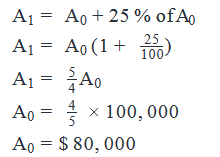Thus, we have inferred the values of A0 and A1 from the question statement.

The question asks us about the percent increase in the tuition fees of University B.

In order to find this percent increase, we need:

(i) Either the values of B0 and B1.

(ii) Or, a relationship of the form B0 = kB1 where k is a constant

Step 3: Analyze statement 1

As per Statement 1,

In the year 2008, the tuition fees of University A was 2.5 times the tuition fees of University B.

A0 = 2.5 (B0)

Since we know the value of A0, from the above equation, we will be able to find the value of B0.

However, this statement provides us no clue about the value of B1 or even about the relationship between B0 and B1.

Thus, Statement 1 is not sufficient.

Step 4: Analyze statement 2

As per Statement 2,

In the year 2012, the tuition fees of University A is \$30,000 more than the tuition fees of University B

A1 = B1 + 30,000

B1 = 100,000 – 30,000

B1 = 70,000

However, Statement 2 gives us no idea about the value of B0 or even about the relationship between B0 and B1.

Therefore, Statement 2 is Not Sufficient.

Step 5: Analyze Both Statements Together (if needed)

From Statement 1:

A0 = 2.5 (B0)

80000 = 2.5 (B0)

Thus,

B0 = 32,000

From Statement 2:

B1 = 70,000

Thus, we now know the value of B0 and B1.

So, we will be able to calculate the percent increase in the tuition fees of University B from 2008 to 2012.

Thus, both the statements together are sufficient to arrive at a unique solution.

Test: Percentages- 1 - Question 11

In her second attempt of a standardized test, Joan scored 20 percent higher than her first attempt. How much did she score in her second attempt?

(1) Joan scored 200 in her first attempt.
(2) The absolute difference between her two scores was 40 units.

Detailed Solution for Test: Percentages- 1 - Question 11

Steps 1 & 2: Understand Question and Draw Inferences

Given that Joan gave two attempts at a standardized test.

Let her score in her first attempt = F.

Given that her score in her second attempt = F + 20% of F = 1.2F

We need to find the value of F to be able to determine her score in the second attempt.

Step 3: Analyze Statement 1

This statement says that Joan scored 200 in her first attempt.

• F = 200

Therefore we can calculate her score in her second attempt = 1.2F

Therefore statement 1 is sufficient to arrive at a unique answer.

Step 4: Analyze Statement 2

This statement says that the absolute difference between her scores in the first and second attempts is 40 units.

• 1.2F – F = 40
• 0.2F = 40
• F = 200.

Since we obtained the value of F, we can calculate the value of 1.2F.

Therefore statement 2 is sufficient to arrive at a unique answer.

Step 5: Analyze Both Statements Together (if needed)

Since we arrived at a unique answer in step 3 and step 4 above, this step is not needed.

Test: Percentages- 1 - Question 12

If Rony’s team played 50% of the total 80 matches conducted in a tournament, what percentage of the matches played by Rony’s team did the team win? (Assume that win and loss are the only possible outcomes. None of the matches ended up in a tie.)

(1) Rony’s team won 60% of the first 30 matches played by them and also won all the remaining matches

(2) The number of matches won by Rony’s team is 133% more than the number of matches lost

Detailed Solution for Test: Percentages- 1 - Question 12

Steps 1 & 2: Understand Question and Draw Inferences

Given that total matches played in the tournament = 80.

Number of matches played by Rony’s team (T) = 50% of 80 = 40.

Let the number of matches won by Rony’s team = W.

We need to find the win percentage of Rony’s team = (W/T)*100 = (W/40) * 100

Therefore we need to find the value of W to calculate the win percentage.

Step 3: Analyze Statement 1

The statement says that Rony’s team won 60% of the first 30 matches and then won all of the remaining 10 matches played by them.

So the number of matches won by Rony’s team = W = (60% of 30) + 10 = 18 + 10 = 28.

We know the value of W and therefore we can calculate the required win percentage of Rony’s team.

Therefore Statement 1 is sufficient to arrive at a unique answer.

Step 4: Analyze Statement 2

The statement says that number of matches won by Rony’s team is 133% more than the number of matches lost by them.
Since the total number of matches is 40, the number of matches lost = (40 – W).

Therefore

W = (40 – W) + 133% of (40 –W)

• W = (40 – W) + (4/3)*(40 – W)

(Note that since 33% is equivalent to 1/3, 133% is equivalent to 4/3)

• 3W = 280 – 7W
• W = 28

Therefore statement 2 is sufficient to arrive at a unique answer.

Step 5: Analyze Both Statements Together (if needed)

We arrived at a unique answer in step 3 and step 4 above. So this step is not needed.

Test: Percentages- 1 - Question 13

Jack made a withdrawal from his savings account to fund a medical emergency.  What was the percent decrease in his savings fund, if his savings fund post withdrawal will need to be increased by 25 percent to be restored to its initial amount?

Detailed Solution for Test: Percentages- 1 - Question 13

Step 1: Question statement and Inferences

Let the withdrawal from the fund be p%

Let the initial amount be X

Balance after withdrawal =  B = X -p% of X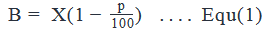Step 2: Finding required values

We are also given that B needs to be increased by 25% to bring it back to X.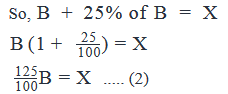Step 3: Calculating the final answer

Substitute Equation (1) in Equation (2):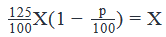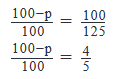5(100−p)=400500–5p=4005p=100p=20%

Thus, the withdrawal from the fund was 20%

Test: Percentages- 1 - Question 14

The number of students in a class is 25, of which 40% are girls. How many more girls must be added in the class such that the percentage of girls is double the current percentage?

Detailed Solution for Test: Percentages- 1 - Question 14

Step 1: Question statement and Inferences

The important thing to keep in mind in this question is that when more girls are added to the class, the total number of students will also increase.

Step 2: Finding required values

Originally:

Number of girls = G  = 40% of 25

G = 40/100 × 25

G = 10

Let x number of girls be added

So, number of girls now =G'  = 10 + x

Total number of students now = 25 + x

Step 3: Calculating the final answer

It’s given that: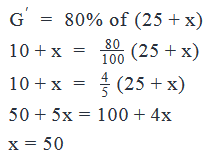Thus, the number of girls added = 50

Looking at the answer choices, we see that Option E is correct

Test: Percentages- 1 - Question 15

Last year country X won 15 gold medals in total 60 competitions. This year country X won only 5 gold medals in a total of 50 competitions. What is the percentage reduction in the percentage of gold medals won per competition this year vs last year?

Detailed Solution for Test: Percentages- 1 - Question 15

Step 1: Question statement and Inferences

We are given that last year country X won 15 gold medals in a total of 60 competitions. However, this year country X won only 5 gold medals in a total of 50 competitions.

We have to find the percentage reduction in the percentage of gold medals won per competition.

Step 2: Finding required values

Now, to calculate the required percentage reduction, we need to first calculate the percentage of gold medals won by country X this year and the similar percentage for last year.

Percentage of gold medals (to competitions) this year = (5/50)*100 = 10%

Percentage of gold medals (to competitions) last year = (15/60)*100 = 25%

We are talking about the reduction in percentage compared to last year.

Therefore the required percentage = [(25 – 10)/25]*100 = 15*4 = 60%

## Quantitative for GMAT

88 videos|99 docs|165 tests
 Use Code STAYHOME200 and get INR 200 additional OFF Use Coupon Code
Information about Test: Percentages- 1 Page
In this test you can find the Exam questions for Test: Percentages- 1 solved & explained in the simplest way possible. Besides giving Questions and answers for Test: Percentages- 1, EduRev gives you an ample number of Online tests for practice

## Quantitative for GMAT

88 videos|99 docs|165 tests